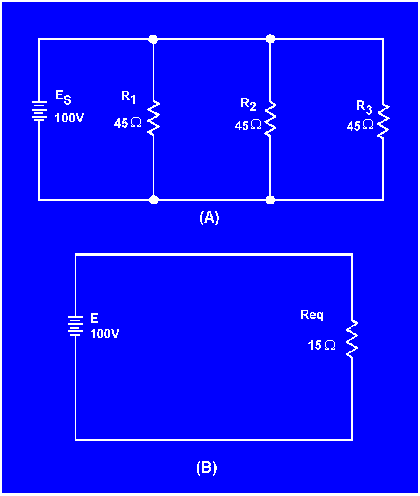voltage source and a single resistor representing total resistance. This process is called reduction to an EQUIVALENT CIRCUIT. Figure 3-49 shows a parallel circuit with three resistors of equal value and the redrawn equivalent circuit."> Equivalent CircuitsCustom SearchPower in a Parallel CircuitMatter, Energy, and ElectricityRules for Parallel DC CircuitsEquivalent Circuits
In the study of electricity, it is often necessary to reduce a complex circuit into a simpler form. Any complex circuit consisting of resistances can be redrawn (reduced) to a basic equivalent circuit containing the voltage source and a single resistor representing total resistance. This process is called reduction to an EQUIVALENT CIRCUIT.

Figure 3-49 shows a parallel circuit with three resistors of equal value and the redrawn equivalent circuit. The parallel circuit shown in part A shows the original circuit. To create the equivalent circuit, you must first calculate the equivalent resistance.

Figure 3-49. - Parallel circuit with equivalent circuit.Given:Solution:Once the equivalent resistance is known, a new circuit is drawn consisting of a single resistor (to represent the equivalent resistance) and the voltage source, as shown in part B.Integrated Publishing, Inc. - A (SDVOSB) Service Disabled Veteran Owned Small Business